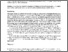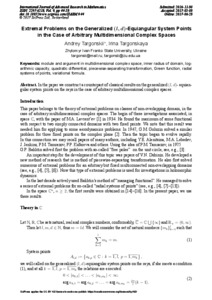Extremal Problems on the Generalized (l; d)-Equiangular System Points in the Case of Arbitrary Multidimensional Complex Spaces

Targonskii, A. L. and Targonskaya, I. I. (2017) Extremal Problems on the Generalized (l; d)-Equiangular System Points in the Case of Arbitrary Multidimensional Complex Spaces. International Journal of Advanced Research in Mathematics, 9. pp. 44-53. ISSN 2297-6213Preview
Text

Abstract

In the paper we construct a counterpart of classical results on the generalized (l; d)- equiangular system points on the rays in the case of arbitrary multidimensional complex spaces

Item Type: Article module and argument in multidimensional complex space, inner radius of domain, logarithmic capacity, quadratic differential, piecewise-separating transformation, Green function, radial systems of points, variational formula Q Science > QA Mathematics > Mathematical Analysis Faculty of Physics and Mathematics > Department of Mathematical Analysis Ірина Ігорівна Таргонська 05 Feb 2018 23:31 05 Feb 2018 23:31 http://eprints.zu.edu.ua/id/eprint/26353View Item